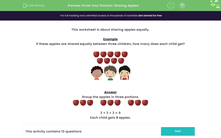# Divide by 2 and 3

In this worksheet, students work out how to share a number of apples equally between two or three people.Key stage:  KS 2

Curriculum topic:   Number: Multiplication and Division

Curriculum subtopic:   Solve Multiplication/Division Problems

Difficulty level:#### Worksheet Overview

In this activity, we will be sharing objects into equal groups to divide by 2 and 3.

Here, we have 6 apples and two children.If we share the apples between the two children, they will each get 3 apples.Let's try an example. This time, we will be sharing into three groups.

Example

If these apples are shared equally between three children, how many does each child get?To share equally, group the apples in three portions.3 + 3 + 3 = 9

Each child gets 3 apples.

### What is EdPlace?

We're your National Curriculum aligned online education content provider helping each child succeed in English, maths and science from year 1 to GCSE. With an EdPlace account you’ll be able to track and measure progress, helping each child achieve their best. We build confidence and attainment by personalising each child’s learning at a level that suits them.

Get started